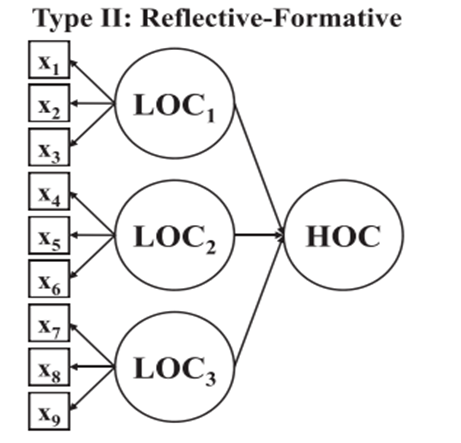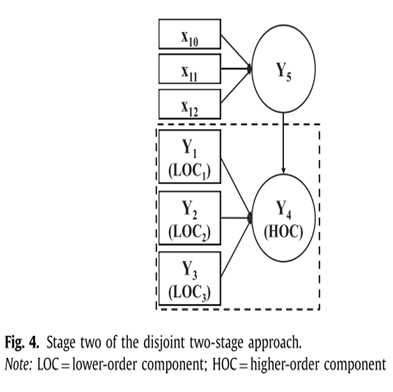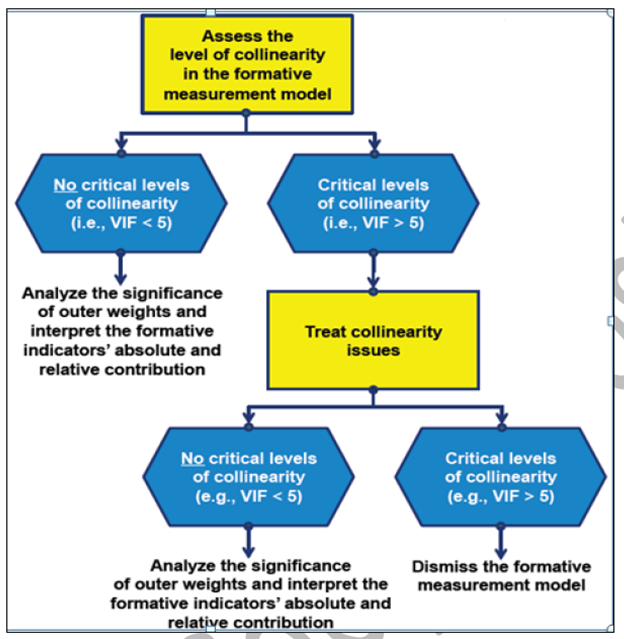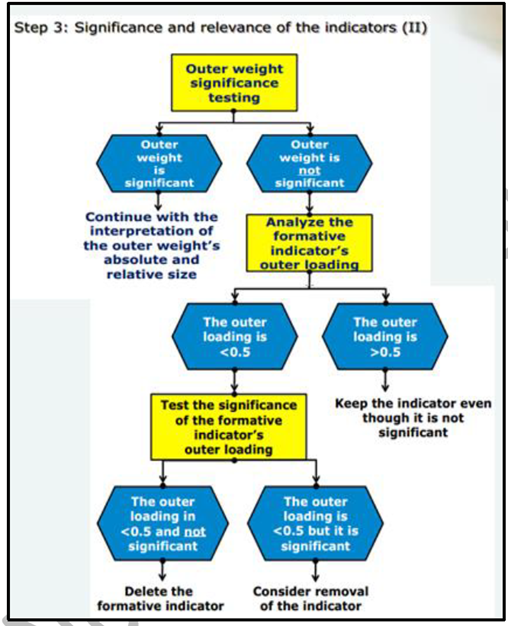# Analyzing and Reporting Reflective-Formative Higher-Order Construct using SMART-PLS

### Analyzing and Reporting Reflective-Formative Higher-Order Construct using SMART-PLS

A Reflective-Formative model is one where the first order has reflective indicators while in the second order the dimensions are formative. Here is how it looks### Two-Stage Approach

Researchers have proposed the two-stage approach as an alternative to the repeated indicators approach.

In fact, research has proposed two versions of the two-stage approach,

1. The embedded two-stage approach and
2. The disjoint two-stage approach, which slightly differ in their model specification in both stages.

For example, while the embedded approach models the entire higher-order construct in its first stage, the disjoint approach initially only draws on the lower-order components (hence the denominations “embedded” and “dis- joint”).

As both versions of the two-stage approach lead to similar results, there is no compelling reason for preferring one over the other. In the following, we introduce first introduce the dis-joint two-stage approach for reflective-formative type higher-order constructs.

### Disjoint Two-Stage Approach

The disjoint two-stage approach differs from the embedded two-stage approach in the specification of both stages.

Rather than using the repeated indicators approach in stage one, the disjoint two-stage approach considers only the lower-order components of the higher-order construct (i.e., without the higher-order component) in the path model.

These are directly linked to all other constructs that the higher-order construct is theoretically related to.

To execute the disjoint two-stage approach, researchers then need to save the construct scores, but only those of the lower order components.

In stage two, these scores are then used to measure the higher-order construct. However, different from the embedded two-stage approach, all other constructs in the path model are estimated using their standard multi-item measures as in stage one (e.g., Y 5 in Fig).### Validating Higher Order Constructs

When evaluating higher-order models, the same model evaluation criteria generally apply as for any PLS-SEM analysis (Chin, 2010b). However, higher-order constructs need to consider two additional measurement models for which the evaluation criteria apply:

1. The measurement models of the lower-order components, and
2. The measurement model of the higher-order construct as a whole, represented by the relationships between the higher-order component and its lower-order components.

### Validating Higher Order Constructs

In stage one, the estimation and measurement model assessment for the lower-order components is based on the standard model, which draws direct relationships between the constructs. The higher-order component is not included in the PLS path model.

Then, in stage two, the latent variable scores from the stage one results allow creating and estimating the model.

The assessment of the stage two results begins with

First, HOCs formative measurement model. The result supports the convergent validity of the higher-order construct, when the path coefficient does not significantly differ from the 0.7 threshold (Hair et al., 2017a).

Second, find that the HOC measurement model is not negatively affected by collinearity and assess the VIF of the LOCs for the HOC.

Finally, Confirm all structural model evaluation results (e.g., significance and relevance for path coefficients, Q², PLS predict).

#### Assess the Collinearity

To validate the higher order constructs, we need to first assess the collinearity